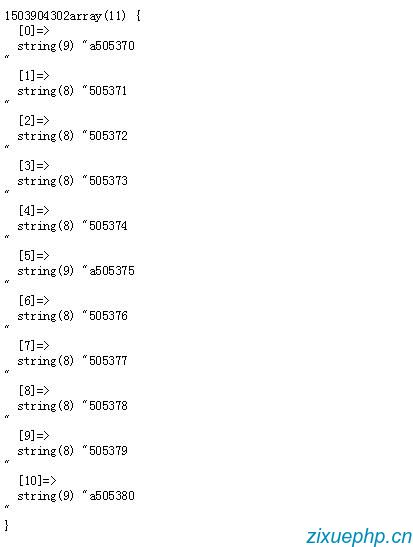# PHP讀取大文件的X行到Y行的內容

PHP真的越來越“面向對象”了，一些原有的基礎的SPL方法都開始陸續地實現出class了。

 //先創建一個有內容的文件// \$stream = fopen("a.txt", "w+");// for(\$i =1; \$i<10000000;\$i++){// \$str = \$i;// if(\$i%5==0){// \$str = 'a'.\$i;// }// file_put_contents("a.txt", \$str."\r\n", FILE_APPEND);// }//再指定行數獲取文件內容function getFileLines(\$filename, \$startLine = 1, \$endLine=50, \$method='rb') {\$content = array();\$count = \$endLine - \$startLine;if(version_compare(PHP_VERSION, '5.1.0', '>=')){// 判斷php版本（因為要用到SplFileObject，PHP>=5.1.0）        \$fp = new SplFileObject(\$filename, \$method);        \$fp->seek(\$startLine-1);// 轉到第N行, seek方法參數從0開始計數            for(\$i = 0; \$i <= \$count; ++\$i) {                \$content[]=\$fp->current();// current()獲取當前行內容                \$fp->next();// 下一行 }}else{//PHP<5.1\$fp = fopen(\$filename, \$method);if(!\$fp) return 'error:can not read file';for (\$i=1;\$i<\$startLine;++\$i) {// 跳過前\$startLine行fgets(\$fp);}for(\$i;\$i<=\$endLine;++\$i){\$content[]=fgets(\$fp);// 讀取文件行內容}fclose(\$fp);}echo time();return array_filter(\$content); // array_filter過濾：false,null,''}var_dump(getFileLines('a.txt',505370，505380));http://blog.csdn.net/u013372487/article/details/51355174

http://php.net/manual/en/class.splfileobject.php• 最新評論
• 總共1條評論2017-08-29 16:57:37 回復

• ? 2014-2016 zixuephp.cn 版權所有
• ICP證：皖ICP備16012107號
• 聯系郵箱：[email protected]
• 交流請加微信：weibobf2899 或掃下方二維碼
•